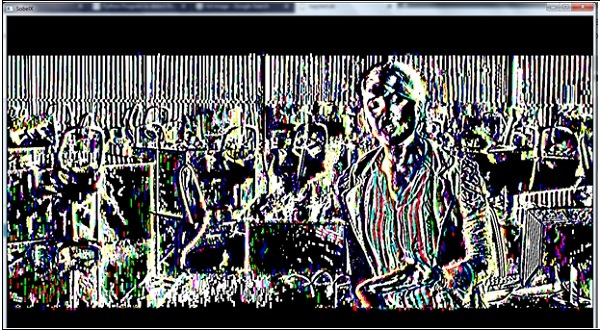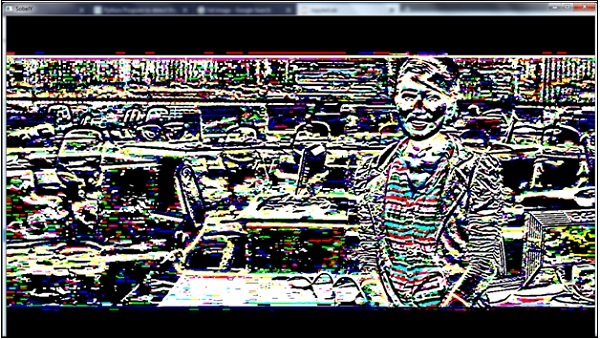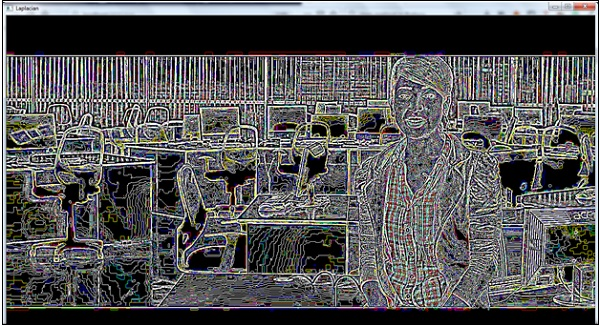# Python Program to detect the edges of an image using OpenCV

PythonProgrammingServer Side Programming

In this problem, we will see how Python can detect edges of an image or video file. To achieve this, we need the OpenCV library. The OpenCV library is mainly designed for computer vision. It is open source. Originally it was designed by Intel. This is free to use under open-source BSD license.

To use the OpenCV functionality, we need to download them using pip.The OpenCV will download the Numpy module. That will also be needed.

sudo pip3 install opencv-python


As input, in this case, we have used one video file. We can also use our webcam to see the real-time effect of this edge detection procedure.

Here we are using a video file. The actual image (frame of the video) is like this −## How the Edge Detection Works?

To detect edges, there are some mathematical observations to check whether the brightness of pixels is changing distinctly.

We need to find the gradient for the grayscale version of our image. In computer vision, when the pixels are transiting from black to white, it is noted as a positive slope. For the white to black transition, it is a negative slope.

## Edge Detection using Derivative of an Image

An image is stored as a matrix, where each element of that matrix holds information about all pixels. To find the derivation we need Laplacian operator. So to get the Laplacian, at first we need the Sobel derivatives. These Sobel derivatives are used to get the gradient variation of an image.

## The Horizontal Sobel Derivative (Sobel X)

This Sobel derivative is obtained through the convolution of the actual image and another matrix (called kernel). The kernel is 3x3 matrix for simple case.

There is a function called sobel(). Using this function, we can find the sobel derivatives. When we are trying to get the Sobel x, then the y part should be 0.

## Example code

import cv2
import numpy as np
capture = cv2.VideoCapture('sample_video.mp4') #Capture frames from video file.
while(capture.isOpened()): # Run the loop until the video ends
ret, frame = capture.read() #Fetch the frames from video
# Convert BGR color to (Hue Saturation Value) mode
hsv_col = cv2.cvtColor(frame, cv2.COLOR_BGR2HSV)
# The Sobelx Method
sobelx = cv2.Sobel(frame,cv2.CV_64F,1,0,ksize=5)
cv2.imshow('SobelX',sobelx)
k = cv2.waitKey(5) & 0xFF
if k == 27: #27 is the ASCII for ESC key. When ESC is pressed, it will stop
break
capture.release()
cv2.destroyAllWindows() #Clean memory after removing the windows


## Output## The Vertical Sobel Derivative (Sobel Y)

Similarly we can find the Vertical Sobel derivative using the sobel() function. In this case the x part will be 0.

## Example code

import cv2
import numpy as np
capture = cv2.VideoCapture('sample_video.mp4') #Capture frames from video file.
while(capture.isOpened()): # Run the loop until the video ends
ret, frame = capture.read() #Fetch the frames from video
# Convert BGR color to (Hue Saturation Value) mode
hsv_col = cv2.cvtColor(frame, cv2.COLOR_BGR2HSV)
# The Sobely Method
sobely = cv2.Sobel(frame,cv2.CV_64F,0,1,ksize=5)
cv2.imshow('SobelY',sobely)
k = cv2.waitKey(5) & 0xFF
if k == 27: #27 is the ASCII for ESC key. When ESC is pressed, it will stop
break
capture.release()
cv2.destroyAllWindows() #Clean memory after removing the windows


## Output## The Laplacian Derivative

At last we will see the Laplacian Derivative of the image. There is a function called Laplacian(). It is used to get the derivative.

## Example code

import cv2
import numpy as np
capture = cv2.VideoCapture('sample_video.mp4') #Capture frames from video file.
while(capture.isOpened()): # Run the loop until the video ends
ret, frame = capture.read() #Fetch the frames from video
# Convert BGR color to (Hue Saturation Value) mode
hsv_col = cv2.cvtColor(frame, cv2.COLOR_BGR2HSV)
# The Laplacian Method
laplacian = cv2.Laplacian(frame,cv2.CV_64F)
cv2.imshow('Laplacian',laplacian)
k = cv2.waitKey(5) & 0xFF
if k == 27: #27 is the ASCII for ESC key. When ESC is pressed, it will stop
break
capture.release()
cv2.destroyAllWindows() #Clean memory after removing the windows


## Output